The proc_means function simulates a SAS® PROC MEANS procedure. It is used to generate summary statistics on numeric variables. The function is both interactive and returns datasets.

## Create Sample Data

Let’s again create some sample data. This sample data is identical to that created for the proc_freq tutorial, but has an additional grouping variable “g”:

# Create sample data
text = 'x y z   g
6 A 60  P
6 A 70  P
2 A 100 P
2 B 10  P
3 B 67  Q
2 C 81  Q
3 C 63  Q
5 C 55  Q')

# View sample data
dat
x y   z g
1 6 A  60 P
2 6 A  70 P
3 2 A 100 P
4 2 B  10 P
5 3 B  67 Q
6 2 C  81 Q
7 3 C  63 Q
8 5 C  55 Q

## Get Summary Statistics

If no parameters are specified, the proc_means function will calculate N, Means, Standard Deviation, Minimum, and Maximum on all numeric variables.

Note that the options statement has been added to pass CRAN checks. When you are running code samples, this statement may be omitted.

# Turn off printing for CRAN checks
options("procs.print" = FALSE)

# No parameters
proc_means(dat)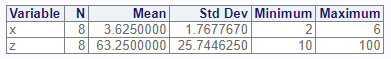## Selected Variables

If you don’t want statistics on all numeric variables, you may specify variables on the var parameter:

# Specific variable
proc_means(dat, var = x)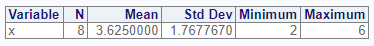## Statistics Options

The proc_means function has a stats parameter that allows you to control which statistics are generated. There are many statistics keywords. Here is a sample of some of the most frequently used keywords:

Keyword Description
N Number of Observations
NMISS Number of missing observations
MEAN Arithmetic mean
STD Standard Deviation
MIN Minimum
MAX Maximum
SUM Sum of observations
MEDIAN 50th percentile
P1 1st percentile
P5 5st percentile
P10 10th percentile
P90 90th percentile
P95 95th percentile
P99 99th percentile
Q1 First Quartile
Q3 Third Quartile

Now that we know some statistics keywords, let’s practice using them. Here is an example which calculates the median, sum, first quartile, and third quartile for all numeric variables in our sample data:

# Custom statistics options
proc_means(dat, stats = v(median, sum, q1, q3))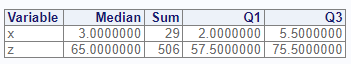## Output Datasets

Similar to the proc_freq function, proc_means can return datasets. There are three options: “out”, “report”, and “none”. The “out” option returns datasets meant for further manipulation and analysis, and is the default. The “report” keyword requests the exact datasets used in the interactive report. Specifying either one of these options will cause the function to return data.

Here is an example that shows the difference in the “report” and “out” options:

# Output dataset using "report" option
res1 <- proc_means(dat,
stats = v(median, sum, q1, q3),
output = report)

# View results
res1
#   VAR MEDIAN SUM   Q1   Q3
# 1   x      3  29  2.0  5.5
# 2   z     65 506 57.5 75.5

# Output dataset using "all" option
res2 <- proc_means(dat,
stats = v(median, sum, q1, q3),
output = out)

# View results
res2
#   TYPE FREQ VAR MEDIAN SUM   Q1   Q3
# 1    0    8   x      3  29  2.0  5.5
# 2    0    8   z     65 506 57.5 75.5

As can be seen in the above example, the “out” dataset includes additional variables for TYPE and FREQ. These additional variables can be turned off with options:


# Turn off TYPE and FREQ variables
res3 <- proc_means(dat,
stats = v(median, sum, q1, q3),
output = all,
options = v(notype, nofreq))

# View results
res3
#   VAR MEDIAN SUM   Q1   Q3
# 1   x      3  29  2.0  5.5
# 2   z     65 506 57.5 75.5

## Grouping

The proc_means function provides two grouping parameters: class and by. These parameters identify a variable or variables for subsetting the input data. While these parameters have similar capabilities, there are some difference between them. The differences can be examined by comparing the two function calls.

### Class

# Class grouping
res1 <- proc_means(dat, stats = v(median, sum, q1, q3),
class = y, options = v(maxdec = 4))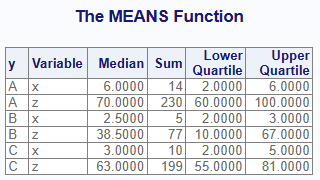Below is the output dataset from the class parameter. Notice that summary values have been provided for each variable, in addition to the subsets by the class variable. The summary rows are identifed by TYPE = 0, while the subset rows are TYPE = 1.

# View results - class
res1
#   CLASS TYPE FREQ VAR MEDIAN SUM   Q1    Q3
# 1  <NA>    0    8   x    3.0  29  2.0   5.5
# 2  <NA>    0    8   z   65.0 506 57.5  75.5
# 3     A    1    3   x    6.0  14  2.0   6.0
# 4     A    1    3   z   70.0 230 60.0 100.0
# 5     B    1    2   x    2.5   5  2.0   3.0
# 6     B    1    2   z   38.5  77 10.0  67.0
# 7     C    1    3   x    3.0  10  2.0   5.0
# 8     C    1    3   z   63.0 199 55.0  81.0

### By

Here is the same analysis using the by parameter instead of the class parameter:

# By grouping
res2 <- proc_means(dat, stats = v(median, sum, q1, q3),
by = y, options = v(maxdec = 4))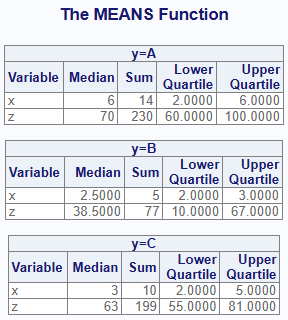Notice that with the by parameter, separate tables are created for each by group on the interactive report.

Now let’s look at the output dataset:

# View results - by
res2
#   BY TYPE FREQ VAR MEDIAN SUM Q1  Q3
# 1  A    0    3   x    6.0  14  2   6
# 2  A    0    3   z   70.0 230 60 100
# 3  B    0    2   x    2.5   5  2   3
# 4  B    0    2   z   38.5  77 10  67
# 5  C    0    3   x    3.0  10  2   5
# 6  C    0    3   z   63.0 199 55  81

The output dataset is also different from the class output. While the TYPE variables exists on the output dataset, the output data for the by group does not include the summary rows. The summary rows are a feature of the class variable. You should select the grouping parameter that most suits your needs.

## Multiple Groups

### Class

The proc_means function can perform analysis with multiple grouping variables. First let’s examine what happens when we pass multiple grouping variables to the class parameter:

# Class grouping - two variables
res1 <- proc_means(dat, stats = v(median, sum, q1, q3),
class = v(g, y), options = v(maxdec = 0))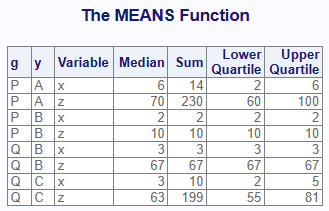Here is the output dataset:

# View results - two class variables
res1
#    CLASS1 CLASS2 TYPE FREQ VAR MEDIAN SUM   Q1    Q3
# 1    <NA>   <NA>    0    8   x    3.0  29  2.0   5.5
# 2    <NA>   <NA>    0    8   z   65.0 506 57.5  75.5
# 3    <NA>      A    1    3   x    6.0  14  2.0   6.0
# 4    <NA>      A    1    3   z   70.0 230 60.0 100.0
# 5    <NA>      B    1    2   x    2.5   5  2.0   3.0
# 6    <NA>      B    1    2   z   38.5  77 10.0  67.0
# 7    <NA>      C    1    3   x    3.0  10  2.0   5.0
# 8    <NA>      C    1    3   z   63.0 199 55.0  81.0
# 9       P   <NA>    2    4   x    4.0  16  2.0   6.0
# 10      P   <NA>    2    4   z   65.0 240 35.0  85.0
# 11      Q   <NA>    2    4   x    3.0  13  2.5   4.0
# 12      Q   <NA>    2    4   z   65.0 266 59.0  74.0
# 13      P      A    3    3   x    6.0  14  2.0   6.0
# 14      P      A    3    3   z   70.0 230 60.0 100.0
# 15      P      B    3    1   x    2.0   2  2.0   2.0
# 16      P      B    3    1   z   10.0  10 10.0  10.0
# 17      Q      B    3    1   x    3.0   3  3.0   3.0
# 18      Q      B    3    1   z   67.0  67 67.0  67.0
# 19      Q      C    3    3   x    3.0  10  2.0   5.0
# 20      Q      C    3    3   z   63.0 199 55.0  81.0

Observe that the function produces statistics for each set of combinations of the class variable. Each level of combinations is identified by the TYPE value. To turn off the class combinations, pass the “nway” option.

### By

Now let’s see what happens when we use the by parameter with two variables:

# By grouping - two variables
res2 <- proc_means(dat, stats = v(median, sum, q1, q3),
by = v(g, y), options = v(maxdec = 0))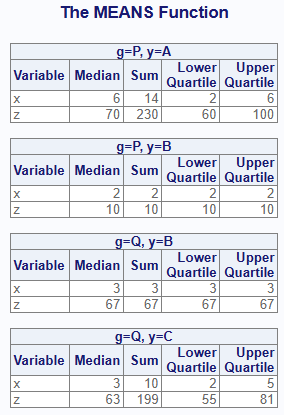Here is the output dataset for the by parameter:

# View results - two by variables
res2
#   BY1 BY2 TYPE FREQ VAR MEDIAN SUM Q1  Q3
# 1   P   A    0    3   x      6  14  2   6
# 2   P   A    0    3   z     70 230 60 100
# 3   P   B    0    1   x      2   2  2   2
# 4   P   B    0    1   z     10  10 10  10
# 5   Q   B    0    1   x      3   3  3   3
# 6   Q   B    0    1   z     67  67 67  67
# 7   Q   C    0    3   x      3  10  2   5
# 8   Q   C    0    3   z     63 199 55  81

### By and Class

Finally, let’s see what happens when we specify both by and class parameters:

# By grouping - by and class
res3 <- proc_means(dat, stats = v(median, sum, q1, q3),
by = g,
class = y,
options = v(maxdec = 0))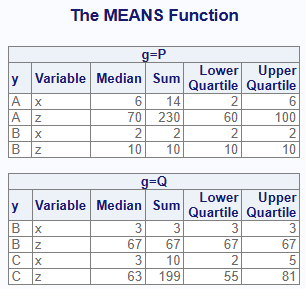# View results - by and class
res3
#    BY CLASS TYPE FREQ VAR MEDIAN SUM   Q1  Q3
# 1   P  <NA>    0    4   x      4  16  2.0   6
# 2   P  <NA>    0    4   z     65 240 35.0  85
# 3   P     A    1    3   x      6  14  2.0   6
# 4   P     A    1    3   z     70 230 60.0 100
# 5   P     B    1    1   x      2   2  2.0   2
# 6   P     B    1    1   z     10  10 10.0  10
# 7   Q  <NA>    0    4   x      3  13  2.5   4
# 8   Q  <NA>    0    4   z     65 266 59.0  74
# 9   Q     B    1    1   x      3   3  3.0   3
# 10  Q     B    1    1   z     67  67 67.0  67
# 11  Q     C    1    3   x      3  10  2.0   5
# 12  Q     C    1    3   z     63 199 55.0  81

## Data Shaping

The proc_means function also offers options for data shaping. The shaping options can reduce the number of transformations needed to get to your target table.

There are three shaping options: “wide”, “long”, and “stacked”. The “wide” option is the default, and places the statistics in columns and variables in rows. The “long” option places statistics in rows and variables in columns. The “stacked” option puts both statistics and variables in rows.

The following example illustrates the differences between these data shaping options:

# Shape wide
res1 <- proc_means(dat, stats = v(median, sum, q1, q3),
output = wide)

# Wide results
res1
#   TYPE FREQ VAR MEDIAN SUM   Q1   Q3
# 1    0    8   x      3  29  2.0  5.5
# 2    0    8   z     65 506 57.5 75.5

# Shape long
res2 <- proc_means(dat, stats = v(median, sum, q1, q3),
output = long)

# Long results
res2
#   TYPE FREQ   STAT    x     z
# 1    0    8 MEDIAN  3.0  65.0
# 2    0    8    SUM 29.0 506.0
# 3    0    8     Q1  2.0  57.5
# 4    0    8     Q3  5.5  75.5

# Shape stacked
res3 <- proc_means(dat, stats = v(median, sum, q1, q3),
output = stacked)

# Stacked results
res3
#   TYPE FREQ VAR   STAT VALUES
# 1    0    8   x MEDIAN    3.0
# 2    0    8   x    SUM   29.0
# 3    0    8   x     Q1    2.0
# 4    0    8   x     Q3    5.5
# 5    0    8   z MEDIAN   65.0
# 6    0    8   z    SUM  506.0
# 7    0    8   z     Q1   57.5
# 8    0    8   z     Q3   75.5

Next: Data Manipulation